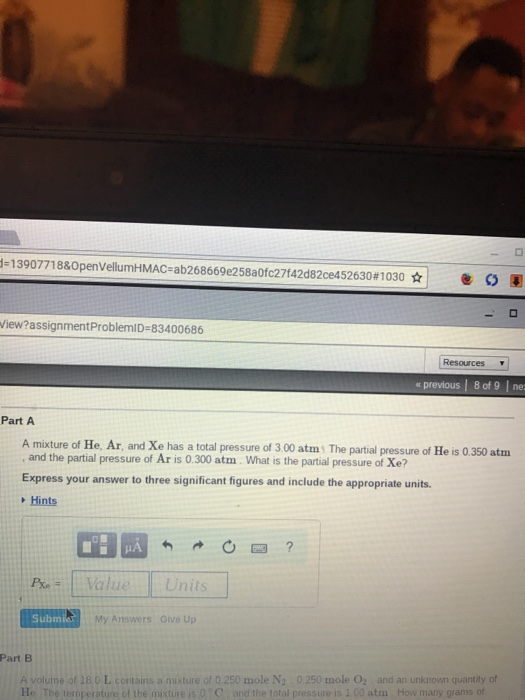# Question & Answer: A mixture of He, Ar and Xe has a total pressure of 3 00 atm. The partial pressure of He is 0 350…..A mixture of He, Ar and Xe has a total pressure of 3 00 atm. The partial pressure of He is 0 350 atm, and the partial pressure of Ar is 0.300 atm. What is the partial pressure of Xe? Express your answer to three significant figures and include the appropriate units. A volume of 18.0 L contains a mixture of 0.25 mole N_2 0.25 mole O_2 and an unknown quantity of He. The temperature of the mixture is 0 degree C and the total pressure is 1.00 atm./How many grams of

A)

total pressure = sum of all partial pressures

Don't use plagiarized sources. Get Your Custom Essay on
Question & Answer: A mixture of He, Ar and Xe has a total pressure of 3 00 atm. The partial pressure of He is 0 350…..
GET AN ESSAY WRITTEN FOR YOU FROM AS LOW AS \$13/PAGE

3.00 atm = p(He) + p(Ar) + p(Xe)

3.00 atm = 0.350 + 0.300 + p(Xe)

p(Xe) = 2.35 atm

B)

1st find the total mol of all the gas

Given:

P = 1.0 atm

V = 18.0 L

T = 0.0 oC

= (0.0+273) K

= 273 K

find number of moles using:

P * V = n*R*T

1 atm * 18 L = n * 0.0821 atm.L/mol.K * 273 K

n = 0.8031 mol

total mol = n(N2) + n(O2) + n(He)

0.8031 = 0.250 + 0.250 + n(He)

n(He) = 0.303 mol

molar mass of He = 4 g/mol

so,

mass of He = n(He)*molar mass

= 0.303 mol * 4 g/mol

= 1.21 g